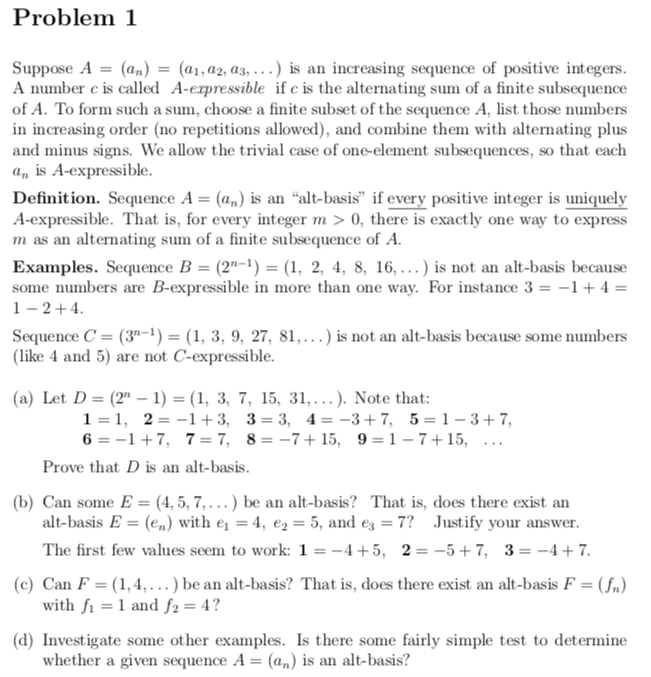全球顶尖数学夏校——罗斯数学训练营（Ross）正式开放申请！申请流程+入营测试

• 数学是理化生及经济学的基础学科，对于不确定未来专业方向的学生来讲申请数学夏校是最稳妥最有效的背景提升方法；
• ROSS、SUMaC、PROMYS等顶尖数学夏校含金量极高，录取的国际学生少之又少，进入这些数学夏校意味着一条腿已经踏进了常青藤；
• 数学夏校一般不强制要求标化成绩，对于数学优异却没有时间刷标化的同学们来讲，更人性化。

The Ross Mathematics Program

Ross课程将会加深学生对数论 (Number Theory) 的理解和运用，为大学本科甚至研究生阶段的数学学习打下坚实基础。通过完成每个课程主题的数学题，学生将熟练掌握数论证明的过程：总结规律、提出假设、探索能证明假设的例子、总结数论、撰写数论证明、完善数论、并探索数论的具体应用。

“简单问题，深度思考”是罗斯训练营的主要理念。

01必须提交

• 官网数学题解答 （一共4题，每题以手写或LaTeX格式作答，需详细描述解题思路和论证过程，以PDF格式提交）
• 1篇个人陈述（字数不限），阐述学生对数学的兴趣、成就和申请项目的原因
• 8篇简短回答（字数不限）, 可参考：https://apply.rossprogram.org/apply/essays
• 高中官方成绩单
• 1篇高中数学老师推荐信
• 英语能力证明（如托福、雅思成绩）

02选择提交

• 奖学金申请
• 简历（以强调数学方面的成就为主）
• 数学相关竞赛奖项2022Ross夏校申请题
Ross营申请材料中，申请题Solution是必须要提交的一项材料，随着申请通道的开放，今年的入营试题也已经公布了↓

※申请题目一览
The number systems Z and Zm (for a positive integer m) were defined in the previous
problem. We also use the notations:
Q is the system of rational numbers.
4Z is the set of multiples of 4 in Z. Similarly for 4Z12.
Consider the following number systems:
Z, Q, 4Z, Z3, Z8, Z9, 4Z12, Z13.
One system may be viewed as similar to another in several different ways.
(a) Let’s measure similarity using algebraic properties. First consider the following
sample properties:
(i) If a2 = 1, then a = ±1.
(ii) If 2x = 0, then x = 0.
(iii) If c2 = 0, then c = 0.
Which of the systems above have properties (i), (ii), and/or (iii)?
(b) Formulate another algebraic property and determine which of those systems have
that property.
Write down some additional algebraic properties and investigate them.
(c) In your opinion, which of the listed systems are “most similar” to each another?